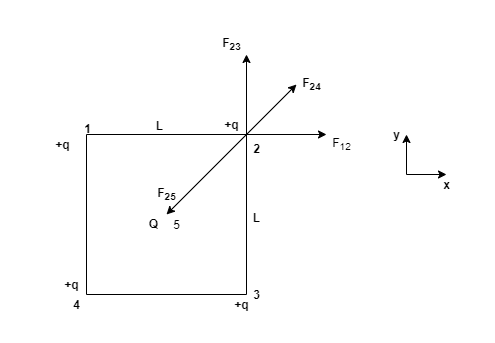# Problem: Four equal positive point charges, each of charge 5.7 µC , are at the corners of a square of side 9.2 cm. What charge should be placed at the center of the square so that all charges are at equilibrium?

###### FREE Expert Solution

Coulomb's law:

$\overline{){\mathbf{F}}{\mathbf{=}}\frac{\mathbf{k}{\mathbf{q}}_{\mathbf{1}}{\mathbf{q}}_{\mathbf{2}}}{{\mathbf{r}}^{\mathbf{2}}}}$diagonal squared = 2L2

F12 = kq2/L2

F23 = kq2/L2

F24 = kq2/(2L2)

$\begin{array}{rcl}{\mathbf{r}}_{\mathbf{25}}& \mathbf{=}& \frac{\sqrt{\mathbf{2}}\mathbf{·}\mathbf{L}}{\mathbf{2}}\\ {\mathbf{\left(}{\mathbf{r}}_{\mathbf{25}}\mathbf{\right)}}^{\mathbf{2}}& \mathbf{=}& \frac{\mathbf{2}{\mathbf{L}}^{\mathbf{2}}}{\mathbf{4}}\\ & \mathbf{=}& \frac{{\mathbf{L}}^{\mathbf{2}}}{\mathbf{2}}\end{array}$

91% (302 ratings)###### Problem Details

Four equal positive point charges, each of charge 5.7 µC , are at the corners of a square of side 9.2 cm.

What charge should be placed at the center of the square so that all charges are at equilibrium?